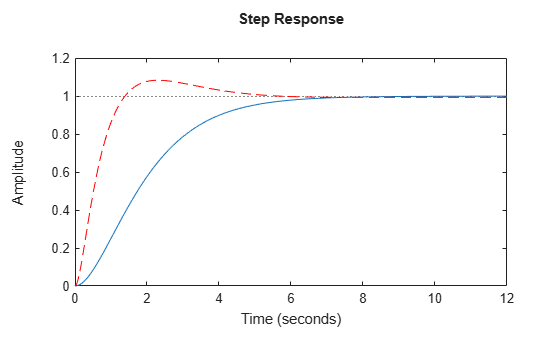# make1DOF

Convert 2-DOF PID controller to 1-DOF controller

## Syntax

``C1 = make1DOF(C2)``

## Description

example

````C1 = make1DOF(C2)` converts the two-degree-of-freedom PID controller `C2` to one degree of freedom by removing the terms that depend on coefficients b and c. ```

## Examples

collapse all

Design a 2-DOF PID controller for a plant.

```G = tf(1,[1 0.5 0.1]); C2 = pidtune(G,'pidf2',1.5)```
```C2 = 1 s u = Kp (b*r-y) + Ki --- (r-y) + Kd -------- (c*r-y) s Tf*s+1 with Kp = 1.12, Ki = 0.23, Kd = 1.3, Tf = 0.122, b = 0.664, c = 0.0136 Continuous-time 2-DOF PIDF controller in parallel form. ```

Convert the controller to one degree of freedom.

`C1 = make1DOF(C2)`
```C1 = 1 s Kp + Ki * --- + Kd * -------- s Tf*s+1 with Kp = 1.12, Ki = 0.23, Kd = 1.3, Tf = 0.122 Continuous-time PIDF controller in parallel form. ```

The new controller has the same PID gains and filter constant. However, `make1DOF` removes the terms involving the setpoint weights `b` and `c`. Therefore, in a closed loop with the plant `G`, the 2-DOF controller `C2` yields a different closed-loop response from `C1`.

```CM = tf(C2); T2 = CM(1)*feedback(G,-CM(2)); T1 = feedback(G*C1,1); stepplot(T2,T1,'r--')```## Input Arguments

collapse all

2-DOF PID controller, specified as a `pid2` object or a `pidstd2` object.

## Output Arguments

collapse all

1-DOF PID controller, returned as a `pid` or `pidstd` object. `C1` is in parallel form if `C2` is in parallel form, and standard form if `C2` is in standard form.

For example, suppose `C2` is a continuous-time, parallel-form 2-DOF `pid2` controller. The relationship between the inputs, r and y, and the output u of `C2` is given by:

`$u={K}_{p}\left(br-y\right)+\frac{{K}_{i}}{s}\left(r-y\right)+\frac{{K}_{d}s}{{T}_{f}s+1}\left(cr-y\right).$`

Then `C1` is a parallel-form 1-DOF `pid` controller of the form:

`${C}_{1}={K}_{p}+\frac{{K}_{i}}{s}+\frac{{K}_{d}s}{{T}_{f}s+1}.$`

The PID gains Kp, Ki, and Kd, and the filter time constant Tf are unchanged. `make1DOF` removes the terms that depend on the setpoint weights b and c. For more information about 2-DOF PID controllers, see Two-Degree-of-Freedom PID Controllers.

The conversion also preserves the values of the properties `Ts`, `TimeUnit`, ```Sampling Grid```, `IFormula`, and `DFormula`.